## Star PolygonA star polygon, withPositive Integers, is a figure formed by connecting with straight lines every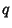th point out of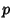regularly spaced points lying on a Circumference. The numberis called the Density of the star polygon. Without loss of generality, take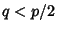.

The usual definition (Coxeter 1969) requiresandto be Relatively Prime. However, the star polygon can also be generalized to the Star Figure (or improper'' star polygon) whenandshare a common divisor (Savio and Suryanaroyan 1993). For such a figure, if all points are not connected after the first pass, i.e., if, then start with the first unconnected point and repeat the procedure. Repeat until all points are connected. For, thesymbol can be factored as(1)

where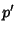(2)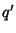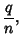(3)

to give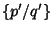figures, each rotated byradians, or.

If, a Regular Polygon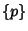is obtained. Special cases ofinclude(the Pentagram),(the Hexagram, or Star of David),(the Star of Lakshmi),(the Octagram),(the Decagram), and(the Dodecagram).

The star polygons were first systematically studied by Thomas Bradwardine.See also Decagram, Hexagram, Nonagram, Octagram, Pentagram, Regular Polygon, Star of Lakshmi, Stellated Polyhedron

References

Coxeter, H. S. M. Star Polygons.'' §2.8 in Introduction to Geometry, 2nd ed. New York: Wiley, pp. 36-38, 1969.

Frederickson, G. Stardom.'' Ch. 16 in Dissections: Plane and Fancy. New York: Cambridge University Press, pp. 172-186, 1997.

Savio, D. Y. and Suryanaroyan, E. R. Chebyshev Polynomials and Regular Polygons.'' Amer. Math. Monthly 100, 657-661, 1993.